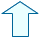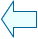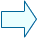The addition and subtraction of fractions Fractions

3. Additions and subtractions of fractions.

When we have both additions and subtractions, we follow the same process as when we have additions only:
In order to add or subtract fractions, they must have the same denominator.
If the fractions have different denominators,  you have to find a common denominator, that is, they are changed  by other equivalent fractions but all  with the same denominator.

In order to do this, follow the following steps:

- First find the least common multiple of the denominators and make it the  denominator of each fraction.

- Next, in order to find each of the new numerators, divide that number by the denominator of a fraction and multiply it by the numerator.

-Finally, add and subtract the numerators and enter the same denominator.

- Simplify if possible.

 In this window you can see the process step by step by clicking on the blue triangle. When you click on Init other fractions will appear. Try to do it several times.Eduardo Barbero CorralSpanish Ministry of Education. Year 2007